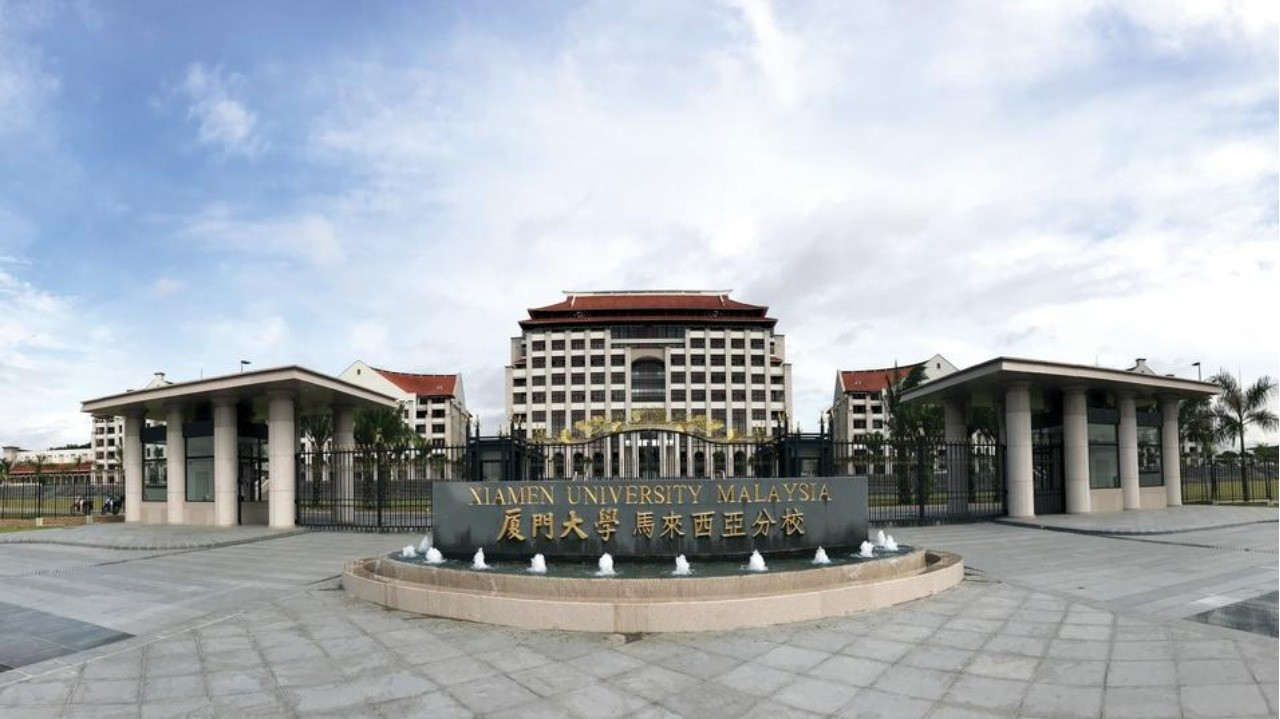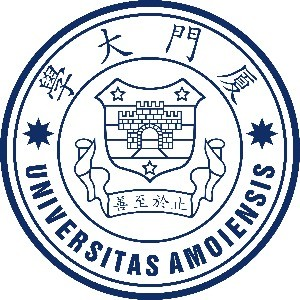# 厦门大学马来西亚分校

## 简介

Master of Science in Mathematics and Applied Mathematics

Xiamen University

Malaysian Qualifications Agency (MQA)

Part-time minimum 3 years / Full-time minimum 2 years

April, September

• 厦门大学马来西亚分校 (雪邦)

## 课程内容

Year 1：Major Core
-Research Methodology

Year 1：Major Elective(8 courses)
-Real Analysis
-Dynamical Systems
-General Topology
-Functional Analysis
-Stochastic Processes
-Regression Analysis
-Time Series
-Combinatorics
-Differentiable Manifolds
-Analytic Number Theory
-Algebraic Number Theory
-Differential Geometry
-Fourier Analysis
-Mathematical Statistics
-Numerical Methods of Partial Differential Equations
-Optimization
-Stochastic Calculus
-Graph Theory

Year 2：Major Core
-Master Dissertation

## 入学资格

-A bachelor's degree in Mathematical Sciences with a minimum CGPA of 2.50 or the equivalent; or
-A bachelor's degree or equivalent with a CGPA below 2.50, can be accepted subject to a minimum of 5 years working experience in a relevant field; or
-A bachelor's degree in Engineering or Other Sciences with a minimum CGPA of 2.75 with at least grade B(Grade point 3.00) in Calculus I(Single Variable Calculus), Calculus II(Multivariable Calculus) and Linear Algebra; or
-A student who does not meet any of the requirements above, but who has had a bachelor's degree with a minimum CGPA of 2.75(in any field), can sit for the examination of Calculus I, Calculus II and Linear Algebra conducted by Xiamen University Malaysia. A student that passes all these three subjects with a grade B(Grade Point 3.00) or above can be admitted into the programme.

## 课程费用

< RM40000 （全课程学杂费预计总额）

RM12,000 per year